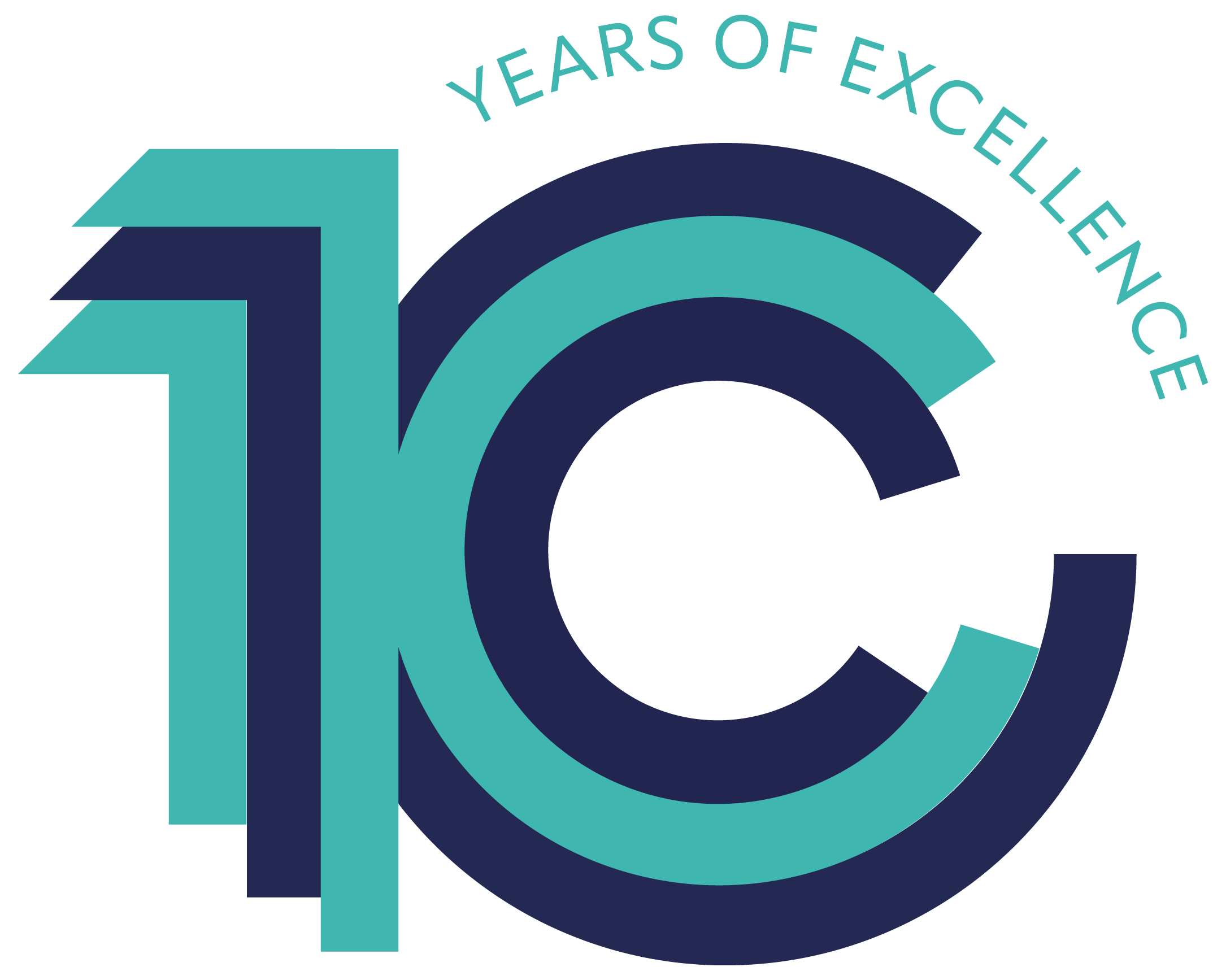## Easy Statistics: Linear and Non-Linear Regression

Duration: Two Days
Price: £595 + VAT
Date: 2021-12-02

## Overview

Learning and applying new statistical techniques can often be a daunting experience. This course is designed to provide participants with a compact and easy to understand introduction to Linear and Non-Linear Regression. It will examine how Ordinary Least Squares (OLS) and how Logit and Probit models work. It will do this without complicated equations but instead focus on visualising underpinning theory. Practical examples are demonstrated. Participants will cover concepts such:

• Bias vs efficiency
• The Gauss-Markov Assumptions
• Interpreting regression results and diagnostic
• Why we need non-linear models

## Intro to Data Visualisation Using ggplot2 in ‘R’

Duration: 1 Day
Price: £390 + VAT
Date: 2022-07-12

## Overview

Data visualisation is an important part of quantitative analysis that allows us to explore data and communicate our findings. ggplot2 is part of the ‘Tidyverse’ family of packages that aim to make data management, analysis and visualisation more user-friendly. It contains a wide range of options to customise plots and can be used for all types of data. This course will discuss good practice when preparing data to plot and introduce a range of commonly used plots within the ggplot package. Users will be shown how to customise plots to convey the message they need in the clearest, most informative way. Please note that this course is only recommended for those that have learned the basics of R elsewhere. The course assumes a basic knowledge of R programming (please get in touch if you’d like information on our ‘Intro to R’ online or face-to-face course).

## Introduction to Regression Analysis Using ‘R’

Duration: Two Half-Days (consecutive)
Price: £490 + VAT
Date: 2022-07-18

## Overview

Regression is a very powerful method of quantitative analysis that allows us to investigate the relationship between an outcome of interest and a number of explanatory variables. From this we can make inferences while accounting for confounding factors and use this to make predictions. This course will introduce the theory behind linear regression analysis and show how R can be used to fit and interpret regression models. Please note that this course is only recommended for those that have learned the basics of R elsewhere. The course assumes a basic knowledge of R programming and statistics (e.g. confidence intervals and p-values). Please get in touch if you’d like information on our ‘Intro to R’ online or face-to-face course.

## Intro to Regression Analysis Using ‘STATA’

Duration: Two Half-Days
Price: £495 + VAT

## Overview

Stata is one of the premier statistical software packages on the market. It is powerful and easy to use. Explore how to perform basic regression analysis in this course including how to build models, interpret output, present output, diagnostic test regression models, introduce complex functional forms and perform hypothesis testing. Concepts will be demonstrated with basic linear regression but many lessons also apply to more complex techniques.

## Introduction to Quant Analysis Using ‘STATA’

Duration: Two Days
Price: £595 + VAT
Date: 2021-10-27

## Overview

This two day course provides a comprehensive introduction to STATA and enables participants to practice application of the issues covered. As a result, delegates will understand (i) the theory behind particular quantitative (mainly econometric) techniques and (ii) how to physically code these into STATA and understand its outputs.